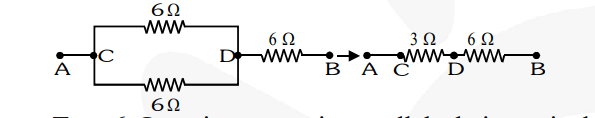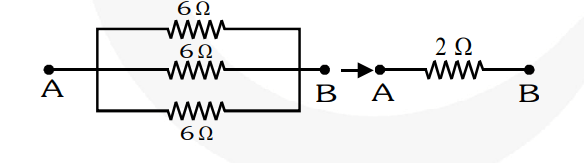# Show how you would connect three resistors,Question.
Show how you would connect three resistors, each of resistance $6 \Omega$, so that the combination has a resistance of

(i) $9 \Omega$

(ii) $2 \Omega$.

solution:

(i) In order to get a resistance of $9 \Omega$ from three resistors, we connect two $6 \Omega$ resistors in parallel and this parallel combination in series with the third $6 \Omega$ resistor as shown in fig.Two $6 \Omega$ resistors are in parallel, their equivalent resistance is,

$\mathrm{R}_{1}=\frac{6 \times 6}{6+6}=\frac{6 \times 6}{12}=3 \Omega$

Now, $R_{1}$ and the third $6 \Omega$ resistors are in series, their equivalent resistance is,

$R_{e}=R_{1}+6=3+6=9 \Omega$

(ii) In order to get a resistance of $2 \Omega$, we connect all the three resistors in parallel as shown in fig. Their equivalent resistance is, $\mathrm{R}_{\mathrm{p}}=\frac{6 \Omega}{3}=2 \Omega$.